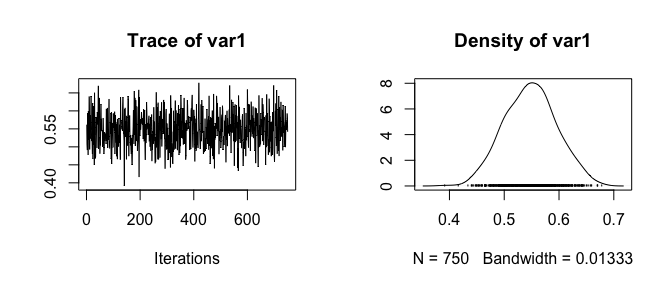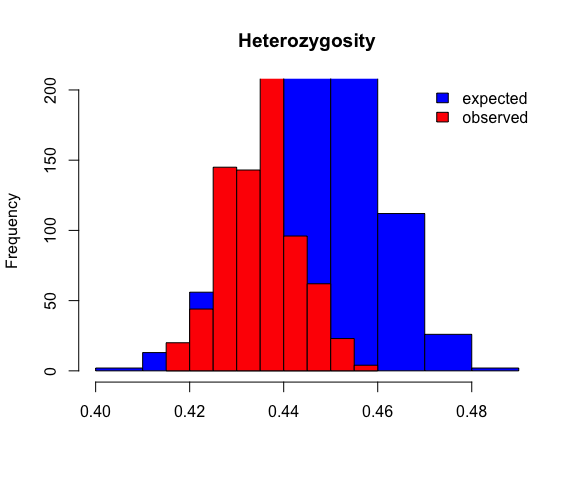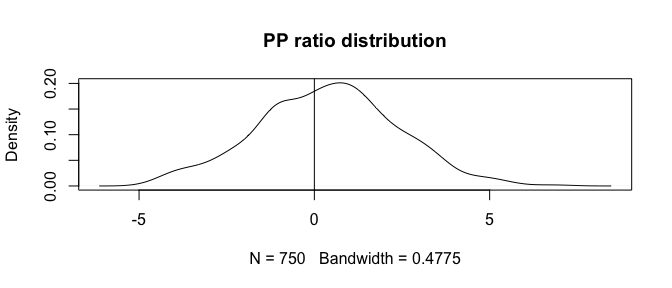# polyfreqs: an R package for Bayesian population genomics in autopolyploids

#### 2016-12-16

polyfreqs is an R package for the estimation of biallelic SNP frequencies, genotypes and heterozygosity (observed and expected; Hardy ) in populations of autopolyploids. It uses a hierarchical Bayesian model to integrate over genotype uncertainty using high throughput sequencing read counts as data (similar to the diploid model of Buerkle and Gompert ). The package implements a Gibbs sampler to draw from the joint posterior distribution of allele frequencies and genotypes. The model is described in our paper in Molecular Ecology Resources Blischak et al.

# 1 Dependencies

polyfreqs uses C++ code to implement its Gibbs sampling algorithm which usually requires the installation of additional software (depending on the operating system being used). Windows users will need to install Rtools. MacOSX users will need to install the Xcode Command Line Tools. Linux users will need an up-to-date version of the GNU C Compiler (gcc), which typically comes installed with most Linux distributions and the r-base-dev package. Looking at the requirements for Rcpp is a good place to start, too.

polyfreqs relies on two other R packages: Rcpp and RcppArmadillo. These are both available on CRAN and can be installed in the usual way using install.packages():

install.packages("Rcpp")
install.packages("RcppArmadillo")

Note that Rcpp and RcppArmadillo also require the compilation of C++ code so make sure that the necessary compilers are installed appropriately for your OS.

# 2 Installation

Installing polyfreqs can be done using the devtools package and the install_github() command. Install devtools using install.packages("devtools"). polyfreqs can then be installed as follows:

devtools::install_github("pblischak/polyfreqs")

# 3 Getting started

## 3.1 Formatting data

polyfreqs takes as input matrices of total and reference read counts and expects that these matrices will have (at a minimum) row names. An optional row of locus names can also be put as the first row. Reading in data from a text file that is in this format is straightforward in R with the read.table() function. An example of what the data should look like is below:

loc1  loc2  loc3  loc4  loc5  ... locL
ind1  23  33  19  22  21  ... 30
ind2  22  19  18  31  29  ... 34
.
.
.
indN  21  17  24  30  28  ... 32

Data formatted in this way can then be read in as follows:

total_table <- read.table("total_read.txt", row.names=1, header=T)
ref_table <- read.table("ref_read.txt", row.names=1, header=T)

If you don’t have a row of locus names on the first line, you can simply remove the header=T argument from the read.table() function.

NB: data run through the polyfreqs() function need to be of class “matrix” so make sure you convert the table objects (total_table and ref_table) using the as.matrix() command before running them through polyfreqs.

## 3.2 Data simulation

We will start by analyzing a data set of simulated read counts for 30 individuals at 5 loci (for the sake of speed—feel free to simulate more loci if you want). We will simulate the total number of reads as a Poisson random variable with mean $$\lambda=15$$ reads per locus per individual. After loading the polyfreqs package using library(polyfreqs) we can simulate data using the following code:

# Simulation setup. Feel free to mess around with these values.
library(polyfreqs)
n_ind <- 30
n_loci <- 5
lambda <- 15
error <- 0.01
allele_freqs <- runif(n_loci, 0.1, 0.9)
ploidy <- 4

dat <- sim_reads(allele_freqs, n_ind, lambda, ploidy, error)

The sim_reads function returns the genotype, total reads and reference reads matrices. We can check out their names and look at what the matrices contain before we move forward and run them through polyfreqs.

names(dat)
##  "genos"        "tot_read_mat" "ref_read_mat"
dat$genos[1:2,1:5] ## [,1] [,2] [,3] [,4] [,5] ## [1,] 1 2 3 3 1 ## [2,] 4 1 4 1 0 dat$tot_read_mat[1:2,1:5]
##      loc1 loc2 loc3 loc4 loc5
## ind1   16   10   12   16   19
## ind2   12   18   11   15   11
dat$ref_read_mat, ploidy=4,iter=itr, thin=thn, outfile=ofile) ## 3.4 MCMC diagnostics using the coda package If you don’t have the coda package installed then you can install it using install.packages("coda"). The code below will read in the output from polyfreqs, convert it to the correct object class for using coda (class mcmc), check that the effective sample sizes (ESS) are greater than 200 (or any other cut-off that you want—just substitute another number) and then prints the names of any loci that have ESS values less than 200. We’ll use a burn-in of 25%, so that means we’ll only use sample 251 through 1000. library(coda) # Read in the MCMC output as a table. p_table<-read.table("polyfreqs_100k-mcmc.out",header=T,row.names=1) # Convert the table to an mcmc object. p_post<-mcmc(p_table[251:1000,]) # check that effective sample sizes are greater than 200 sum(effectiveSize(p_post) < 200) ##  0 # If any are less than 200, we can see which ones they are colnames(p_post[,effectiveSize(p_post) < 200]) ## NULL By default coda will produce a trace plot and a density plot for any individual allele frequency parameter (which is just a column in the p_post object) using the regular plot command. plot(p_post[,1])All trace plots can be examined successively as well using the traceplot command. The following code will allow you to flip through the trace plots for all allele frequency parameters by pressing Enter (we only have 5 here but you may have more if you simulated more loci). par(ask=T) traceplot(p_post) # Remember to reset the ask plotting parameter to FALSE. par(ask=F) ## 3.5 Heterozygosity We measure heterozygosity on a per locus basis using the unbiased estimators from Hardy (2015). They are returned automatically by the polyfreqs() function as het_obs and het_exp. To get a multi-locus estimate, the mean can be taked across loci and then the posterior distributions of expected and observed heterozygosity can be compared. # Take the mean across loci using the apply function. # Take 25% burn-in as well multi_het_obs <- apply(out2$het_obs[251:1000,],1,mean)
multi_het_exp <- apply(out2$het_exp[251:1000,],1,mean) # Check for convergence of the multi-locus estimate effectiveSize(multi_het_obs) ## var1 ## 750 effectiveSize(multi_het_exp) ## var1 ## 750 # Plot histograms to visually compare the estimates hist(multi_het_exp, col="blue", main="Heterozygosity", xlab="", ylim=c(0,200)) hist(multi_het_obs, col="red", add=T) legend(x="topright", c("expected","observed"), col=c("blue","red"), fill=c("blue","red"), bty="n")# Gets means and 95% highest posterior density (HPD) intervals list("mean_exp" = mean(multi_het_exp), "95HPD_exp" = quantile(multi_het_exp, c(0.025, 0.975)), "mean_obs" = mean(multi_het_obs), "95HPD_obs" = quantile(multi_het_obs, c(0.025, 0.975))) ##$mean_exp
##  0.4485403
##
## $95HPD_exp ## 2.5% 97.5% ## 0.4213813 0.4708124 ## ##$mean_obs
##  0.4354667
##
## $95HPD_obs ## 2.5% 97.5% ## 0.4200000 0.4511111 ## 3.6 Checking model adequacy Assessing model adequacy is an important part of any analysis and is easy to do for a polyfreqs run. The main probability distribution use is the Binomial, so generating new samples is straightforward. If you don’t already have the output from the polyfreqs run read in from the previous step, make sure that you start by reading it in as a table. Next, we’ll run the polyfreqs_pps() function using our total reads (dat$tot_read_mat) and reference reads (dat$ref_read_mat) data. We’ll also use a burn-in of 25% again. # Read in the table using the code below if you haven't already done so. # p_table <- read.table("polyfreqs_100k-mcmc.out", header=T, row.names=1) pps <- polyfreqs_pps(as.matrix(p_table[251:1000,]), dat$tot_read_mat,
dat$ref_read_mat, ploidy, error) names(pps) ##  "ratio_diff" "locus_fit" plot(density(pps$ratio_diff[,1]), main="PP ratio distribution")
abline(v=0)A biological side note

Polyploids are notoriously difficult to work with because of how their chromosomes are inherited. The model in polyfreqs assumes that all loci undergo polysomic inheritance, meaning that all alleles at a each locus are freely exchangeable among individuals in a population and thus are equally likely to be passed on to the next generation. Another way to think about this is that the model basically assumes that genotypes are drawn from a single allele pool per locus. If this isn’t the case, then it would nice to know that. Doing posterior predictive simulations based on the output from a polyfreqs analysis is a nice way of testing the adequacy of a model of polysomic inheritance on a per locus basis.

## 3.7 Parallelization

The model for allele frequencies that is implemented in polyfreqs makes the assumption that loci are independent. Because of this, we can split up the data matrices (total and reference read counts) by columns any way that we want and can run them in parallel on separate nodes of a computing cluster. To facilitate this process, the polyfreqs function provides a function for adding a run specific column tag when printing the MCMC samples: col_header. For example, if you had 100,000 loci that needed to be analyzed and also had access to a computing cluster where you could submit jobs to 100 nodes, then it would be to your advantage to run 100 analyzes of 1,000 loci at one time instead of running all 100,000 loci in a single analysis.

The way that the polyfreqs function does this is by allowing you to specify a string that is associated with each run after you split up the matrices. The typical header for columns printed by polyfreqs is ‘p__loc1’, ‘p__loc2’, etc. So if you have split your original full data set into 10 smaller ones, you can run each one with a unique column header tag so that when they are all brought back together there isn’t any confusion with column names. The simplest way to add the column tags would be to use terms like ‘run1’, ‘run2’, …, but they can be anything that you want.

# Example of running polyfreqs with a 10,000 locus matrix that is split in half
# and uses the col_header parameter to distinguish between runs.

polyfreqs(dat$tot_read_mat[,1:5000], dat$ref_read_mat[,1:5000],
col_header="run1")

polyfreqs(dat$tot_read_mat[,5001:10000], dat$ref_read_mat[,5001:10000],
col_header="run2")

References

• Blischak PD, Kubatko LS, Wolfe AD. 2016. Accounting for genotype uncertainty in the estimation of allele frequencies in autopolyploids. Molecular Ecology Resources 16: 742–754.

• Buerkle CA and Gompert Z. 2013. Population genomics based on low coverage sequencing: how low should we go? Molecular Ecology, 22, 3028–3035.

• Hardy, OJ. 2015. Population genetics of autopolyploids under a mixed mating model and the estimation of selfing rate. Molecular Ecology Resources, doi: 10.1111/1755-0998.12431.